# NCERT Solutions for Class 7 Maths Chapter 3 Data Handling Ex 3.2

NCERT Solutions for Class 7 Maths Chapter 3 Data Handling Ex 3.2

### NCERT Solutions for Class 7 Maths Chapter 3 Data Handling Ex 3.2

NCERT Solutions for Class 7 Maths Chapter 3 Data Handling Exercise 3.2
Ex 3.2 Class 7 Maths Question 1.
The scores in mathematics test (out of 25) of 15 students is as follows:
19, 25, 23, 20, 9, 20, 15, 10, 5, 16, 25, 20, 24, 12, 20
Find the mode and median of this data. Are they same?
Solution:
Given data:
19, 25, 23, 20, 9, 20, 15, 10, 5, 16, 25, 20, 24, 12, 20
Let us arrange the given data in increasing order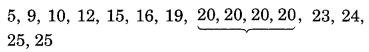Since 20 occurs 4 times (highest)
∴ Mode = 20
n = 15 (odd)= 8th term = 20
Thus, median = 20 and mode = 20
∴ Mode and median are same.

Ex 3.2 Class 7 Maths Question 2.
The runs scored in a cricket match by 11 players is as follows:
6, 15, 120, 50, 100, 80, 10, 15, 8, 10, 15
Find the mean, mode and median of this data. Are the three same?
Solution:
Given data:
6, 15, 120, 50, 100, 80, 10, 15, 8, 10, 15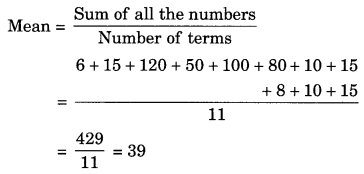Arranging the given data in increasing order, we getHere, 15 occurs 3 times (highest)
∴ Mode = 15
n = 11 (odd)Thus mean = 39, mode = 15 and median = 15
No, they are not same.

Ex 3.2 Class 7 Maths Question 3.
The weights (in kg) of 15 students of a class are:
38, 42, 35, 37, 45, 50, 32, 43, 43, 40, 36, 38, 43, 38, 47
(i) Find the mode and median of this data.
(ii) Is there more than one mode?
Solution:
Given data: 38, 42, 35, 37,45, 50, 32,43, 43,40, 36, 38, 43, 38, 47
Arranging in increasing order, we get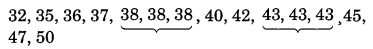(i) Here, 38 and 42 occur 3 times (highest)
Thus mode = 38 and 43
n = 15(odd)(ii) Yes, the given data has two modes i.e. 38 and 43.

Ex 3.2 Class 7 Maths Question 4.
Find the mode and median of the data:
13, 16, 12, 14, 19, 12, 14, 13, 14
Solution:
Arranging the given data in increasing order, we get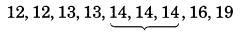Here, 14 occur 3 times (highest)
Thus, mode = 14
n = 9(odd)Ex 3.2 Class 7 Maths Question 5.
Tell whether the statement is true or false.
(i) The mode is always one of the number in a data.
(ii) The mean is one of the numbers in a data.
(iii) The median is always one of the numbers in a data.
(iv) The data 6, 4, 3, 8, 9, 12, 13, 9 has mean 9.
Solution:
(i) True
(ii) False
(iii) True
(iv) False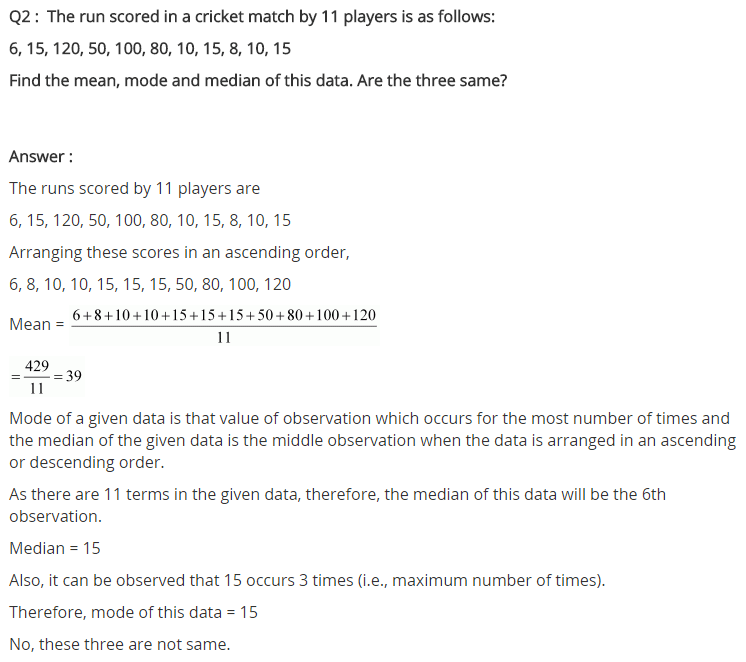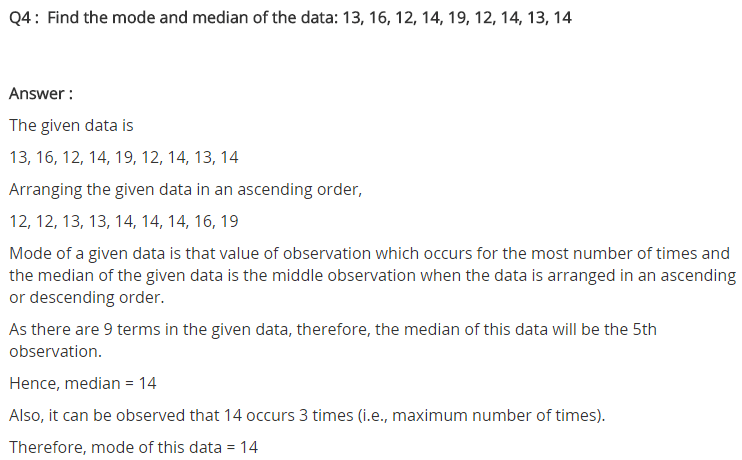## SabDekho

The Complete Educational Website